Math Calculators, Lessons and Formulas

It is time to solve your math problem

mathportal.org
Applications Of The Definite Integrals: (lesson 1 of 3)

## Area Between Two Curves

The area between the curves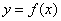and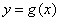between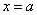and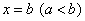is given by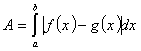.

Example 1:

Find the area of the region bounded by the graphs of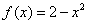and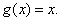Solutions: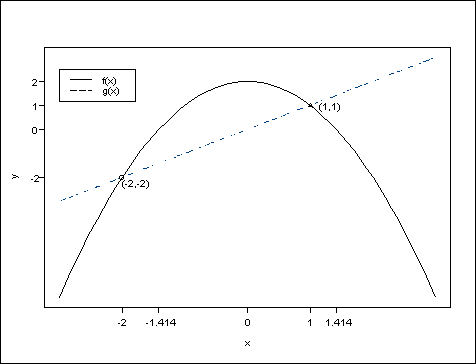The two lines intersect at the points (-2, -2) and (1, 1) since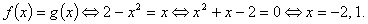Thus, the area isExample 2:

Find the area of the region bounded by the graphs of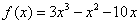and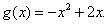Solution: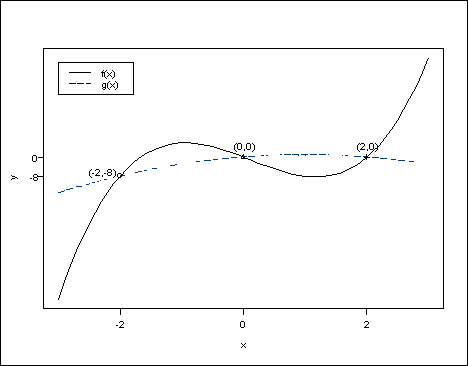The two lines intersect at the points ( -2, -8), ( 0, 0) and ( 2, 0) since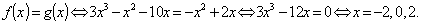Thus, the area is